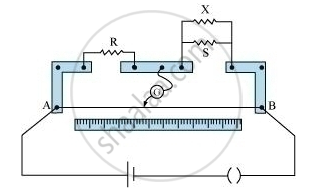PUC Karnataka Science Class 12Department of Pre-University Education, Karnataka
Share

# In a Meter Bridge, the Null Point is Found at a Distance of L1 Cm from A. If Now a Resistance of X is Connected in Parallel with S, the Null Point Occurs at L2 Cm. Obtain a Formula for X in Terms - PUC Karnataka Science Class 12 - Physics

#### Question

In a meter bridge, the null point is found at a distance of l1 cm from A. If now a resistance of X is connected in parallel with S, the null point occurs at l2 cm. Obtain a formula for X in terms of l1l2 and S.#### Solution

Initially, when X is not connected

R/S = l_1/(100 -l_1) [Condition for balance ] ....... (1)

The equivalent resistance (Req) of the combination of X and S is

1/R_(eq) = 1/X +1/S

R_(eq) = (SX)/(X+S)

R/R_(eq) = (l_2)/(100 - l_2)

(R(X+S))/(SX) = (l_2)/((100 - l_2))   ........ (2)

On dividing (i) by (ii), we obtain

R/(R(X +S)) xx (SX)/X  = (l_1(100 - l_2))/(l_2(100 - l_1))

X /(X +S) = (l_1(100 - l_2))/(l_2(100-l_1)

Xl_2 (100 - l_1) = (X +S)l_1(100 - l_2)

XI_2 (100 - l_1) = Xl_1(100 - l_2) + Sl_1 (100 - l_2)

X = (Sl_1 (100 - l_2))/([l_2(100 -l_1) - l_1 (100 - l_1)])

This is the expression for X in terms of Sl1 and l2.

Is there an error in this question or solution?

#### Video TutorialsVIEW ALL 

Solution In a Meter Bridge, the Null Point is Found at a Distance of L1 Cm from A. If Now a Resistance of X is Connected in Parallel with S, the Null Point Occurs at L2 Cm. Obtain a Formula for X in Terms Concept: Metre Bridge.
S